# NCERT Solutions (Exercise 1.2) - Relations and Functions JEE Notes | EduRev

## Commerce : NCERT Solutions (Exercise 1.2) - Relations and Functions JEE Notes | EduRev

The document NCERT Solutions (Exercise 1.2) - Relations and Functions JEE Notes | EduRev is a part of the Commerce Course Mathematics (Maths) Class 12.
All you need of Commerce at this link: Commerce

RELATIONS AND FUNCTIONS

Q.1. Show that the function f: R → R defined by f(x) = 1/x is one-one and onto, where R is the set of all non-zero real numbers. Is the result true, if the domain R is replaced by N with co-domain being same as R?
Ans. It is given that f: RR is defined by f(x)= 1/x
For one – one:
Let x, y ∈ R such that f(x) = f(y)
⇒ 1/x = 1/y
⇒ x = y
∴ f is one – one.
For onto:
It is clear that for y ∈ R, there exists x= 1/y ∈ R [as y ≠ 0] such that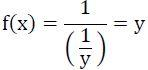Thus, the given function f is one – one and onto.
Now, consider function g: N → R defined by g(x)= 1/x
We have,
g(x1) = g(x2)
⇒ 1/x1 = 1/x2 ⇒ x1 = x2
∴ g is one – one.
Further, it is clear that g is not onto as for 1.2 ∈ R there does not exit any x in N such that g(x)=1/1.2.
Hence, function g is one-one but not onto.

Q.2. Check the injectivity and surjectivity of the following functions:
(i) f : N → N given by f(x) = x2
(ii) f : Z → Z given by f(x) = x2
(iii) f : R → R given by f(x) = x2
(iv) f : N → N given by f(x) = x3
(v) f : Z → Z given by f(x) = x3

Ans.
(i) f: N → N is given by f(x) = x2
It is seen that for x, y ∈ N, f(x) = f(y) ⇒ x= y⇒ x = y.
∴ f is injective.
Now, 2 ∈ N. But, there does not exist any x in N such that f(x) = x2 = 2.
∴ f is not surjective.
Hence, function f is injective but not surjective.
(ii) f: Z → Z is given by f(x) = x2
It is seen that f(−1) = f(1) = 1, but −1 ≠ 1.
∴ f is not injective.
Now, −2 ∈ Z. But, there does not exist any element x ∈ Z such that
f(x) = −2 or x2 = −2.
∴ f is not surjective.
Hence, function f is neither injective nor surjective.
(iii) f: R → R is given by f(x) = x2
It is seen that f(−1) = f(1) = 1, but −1 ≠ 1.
∴ f is not injective.
Now, −2 ∈ R. But, there does not exist any element x ∈ R such that
f(x) = −2 or x2 = −2.
∴ f is not surjective.
Hence, function f is neither injective nor surjective.
(iv) f: N → N given by f(x) = x3
It is seen that for x, y ∈ N, f(x) = f(y) ⇒ x3 = y3 ⇒ x = y.
∴ f is injective.
Now, 2 ∈ N. But, there does not exist any element x ∈ N such that
f(x) = 2 or x3 = 2.
∴ f is not surjective
Hence, function f is injective but not surjective.
(v) f: Z → Z is given by f(x) = x3
It is seen that for x, y ∈ Z, f(x) = f(y) ⇒ x3 = y⇒ x = y.
∴ f is injective.
Now, 2 ∈ Z. But, there does not exist any element x ∈ Z such that
f(x) = 2 or x3 = 2.
∴ f is not surjective.
Hence, function f is injective but not surjective.

Q.3. Prove that the Greatest Integer Function f: R → R given by f(x) = [x], is neither one – one nor onto, where [x] denotes the greatest integer less than or equal to x.
Ans.
f: R → R is given by, f(x) = [x]
It is seen that f(1.2) = [1.2] = 1, f(1.9) = [1.9] = 1.
∴ f(1.2) = f(1.9), but 1.2 ≠ 1.9.
∴ f is not one – one.
Now, consider 0.7 ∈ R.
It is known that f(x) = [x] is always an integer. Thus, there does not exist any element x ∈ R such that f(x) = 0.7.
∴ f is not onto.
Hence, the greatest integer function is neither one – one nor onto.

Q.4. Show that the Modulus Function f: R → R given by f(x) = |x|, is neither one – one nor onto, where |x| is x, if x is positive or 0 and |x| is − x, if x is negative.
Ans.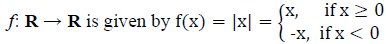It is clear that f(-1) = |-1| = 1 and f(1) = |1| = 1
∴ f(−1) = f(1), but −1 ≠ 1.
∴ f is not one – one. Now, consider −1 ∈ R.
It is known that f(x) = |x| is always non-negative. Thus, there does not exist any element x in domain R such that f(x) = |x| = −1.
∴ f is not onto.
Hence, the modulus function is neither one-one nor onto.

Q.5. Show that the Signum Function f: R → R, given by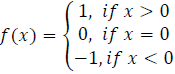is neither one-one nor onto.
Ans.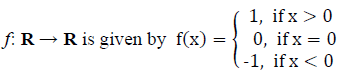It is seen that f(1) = f(2) = 1, but 1 ≠ 2.
∴ f is not one – one.
Now, as f(x) takes only 3 values (1, 0, or −1) for the element −2 in co-domain R, there does not exist any x in domain R such that f(x) = −2.
∴ f is not onto.
Hence, the Signum function is neither one – one nor onto.

Q.6. Let A = {1, 2, 3}, B = {4, 5, 6, 7} and let f = {(1, 4), (2, 5), (3, 6)} be a function from A to B. Show that f is one – one.
Ans. It is given that A = {1, 2, 3}, B = {4, 5, 6, 7}.
f: A → B is defined as f = {(1, 4), (2, 5), (3, 6)}.
∴ f (1) = 4, f (2) = 5, f (3) = 6
It is seen that the images of distinct elements of A under f are distinct.
Hence, function f is one – one.

Q.7. In each of the following cases, state whether the function is one – one, onto or bijective. Justify your answer.
(i) f : R → R defined by f(x) = 3 − 4x
(ii) f : R → R defined by f(x) = 1 + x2

Ans.
(i) f: R → R is defined as f(x) = 3 − 4x.
Let x1, x∈ R such that f(x1) = f(x2)
⇒ 3 - 4x= 3 - 4x⇒ -4x= -4x2
⇒ x1 = x2
∴ f is one – one.
For any real number (y) in R, there exists 3-y/4 in R such that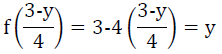∴ f is onto.
Hence, f is bijective.
(ii) f : R → R is defined as f(x) = 1 + x2
Let x1, x∈ R such that f (x1) = f(x2)
⇒ 1+ x12= 1+ x22⇒ x1= x22
⇒ x1 = ± x2
∴ f(x1) = f(x2) does not imply that x1 = x2
For example, f(1)= f(-1)=2
∴ f is not one – one.
Consider an element −2 in co-domain R.
It is seen that f(x) = 1 + x2 is positive for all x ∈ R.
Thus, there does not exist any x in domain R such that f(x) = −2.
∴ f is not onto.
Hence, f is neither one – one nor onto.

Q.8. Let A and B be sets. Show that f: A × B → B × A such that (a, b) = (b, a) is bijective function.
Ans. f : A × B → B × A is defined as f(a, b) = (b, a).
Let (a1, b1), (a2, b2) ∈ A × B such that f(a1, b1) = f(a2, b2)
⇒ (b1, a1) = (b2, a2)
⇒ b= b2 and a= a2
∴ f is one – one.
Now, let (b, a) ∈ B × A be any element.
Then, there exists (a, b) ∈ A × B such that f(a, b) = (b, a). [By definition of f]
∴ f is onto.
Hence, f is bijective.

Q.9. Let f: N → N be defined by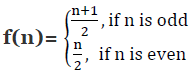for all n ∈ N. State whether the function f is bijective. Justify your answer.
Ans.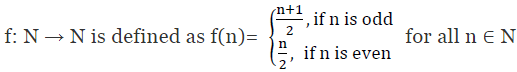It can be observed that:
f(1) = 1 + 1/2 = 1 and f(2) = 2/2 = 1 [By definition of f(n)]
f(1) = f(2), where 1 ≠ 2
∴ f is not one-one.
Consider a natural number (n) in co-domain N.

Case I: n is odd
∴ n = 2r + 1 for some r ∈ N. Then, there exists 4r + 1∈ N such that f(4r + 1) = 4r + 1 + 1/2 = 2r + 1

Case II: n is even
∴ n = 2r for some r ∈ N. Then, there exists 4r ∈ N such that f(4r) = 4r/2 = 2r
∴ f is onto.
Hence, f is not a bijective function.

Q.10. Let A = R − {3} and B = R − {1}. Consider the function f: A → B defined by (x) = (x-2/x-3). Is f one-one and onto? Justify your answer.
Ans.
A = R − {3}, B = R − {1} and f: A → B defined by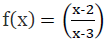Let x, y ∈ A such that f(x) = f(y)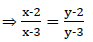⇒ (x – 2)(y – 3) = (y – 2)(x – 3)
⇒ xy – 3x – 2y + 6 = xy – 2x – 3y + 6
⇒ – 3x – 2y = – 2x – 3y ⇒ x = y
∴ f is one-one.
Let y ∈ B = R − {1}. Then, y ≠ 1.
The function f is onto if there exists x ∈ A such that f(x) = y.
Now, f(x) = y
y = (x - 2/ x - 3)
⇒ x – 2 = xy – 3y ⇒ x(1 – y) = – 3y + 2
⇒ x=(2-3y )/(1-y) ∈A [y ≠ 1]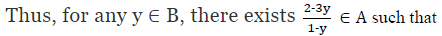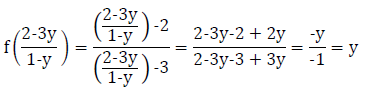∴ f is onto.
Hence, function f is one – one and onto.

Q.11. Let f: R → R be defined as f(x) = x4. Choose the correct answer.
(a) f is one-one onto
(b) f is many-one onto
(c) f is one-one but not onto
(d) f is neither one-one nor onto

Ans. (d)
Solution: f : R → R is defined as f(x) = x4.
Let x, y ∈ R such that f(x) = f(y).
⇒ x4 = y4
⇒ x = ± y
∴ f(x) = f(y) does not imply that x = y.
For example f(1) = f(–1) = 1
∴ f is not one-one.
Consider an element 2 in co-domain R. It is clear that there does not exist any x in domain R such that f(x) = 2.
∴ f is not onto.
Hence, function f is neither one – one nor onto.

Q.12. Let f: R → R be defined as f(x) = 3x. Choose the correct answer.
(a) f is one – one and onto
(b) f is many – one and onto

(c) f is one – one but not onto
(d) f is neither one – one nor onto

Ans. (a)
Solution:
f : R → R is defined as f(x) = 3x.
Let x, y ∈ R such that f(x) = f(y).
⇒ 3x = 3y
⇒ x = y
∴ f is one-one.
Also, for any real number(y) in co-domain R, there exists y/3 in R such that f(y/3) = 3(y/3) = y
∴ f is onto.
Hence, function f is one – one and onto.

Offer running on EduRev: Apply code STAYHOME200 to get INR 200 off on our premium plan EduRev Infinity!

## Mathematics (Maths) Class 12

209 videos|231 docs|124 tests

,

,

,

,

,

,

,

,

,

,

,

,

,

,

,

,

,

,

,

,

,

;